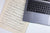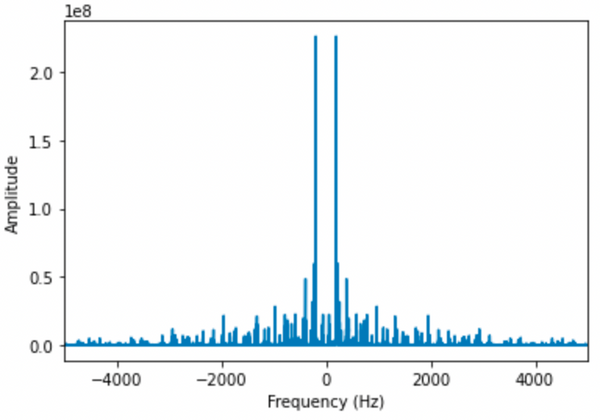# Using Python and Fourier transforms to automate writing sheet music for sound files

Featuring my questionable guitar skills.by Mojan Benham

2 years ago

## Two-line summary

The Fourier transform is a mathematical function that converts waves in the time domain to the frequency domain. With the use of a sliding filter, this post walks through the Python code to conduct a time-frequency analysis of a song in order to convert it to musical notes.

The following post requires an understanding of undergraduate-level calculus and basic Python skills. The reader should be well-versed in advanced functions, integrals, for loops and numpy arrays. Prior knowledge of Fourier or Gabor transforms is not necessary.

For those interested in gaining a deeper theoretical understanding, I would strongly recommend Chapter 13 of Data-Driven Modeling & Scientific Computation as well as the accompanying lecture by the author, J. Nathan Kutz. I cannot speak more highly of Professor Kutz; his engaging content and published works are primarily what inspired this tutorial.

For my coding environment I used Python 3.9.6 with Visual Studio Code notebooks (documentation here). Any Python environment is fine as long as the version is compatible with the libraries used below and is able to print and plot data.

All code used in this post is available on my public github repo, to which I will make cell references throughout so that you can follow along line-by-line.

Cell 1 imports all necessary libraries used hereafter. The libraries must be installed before importing, which I did using pip3 installation. For example, to install matplotlib, I simply ran !pip3 install matplotlib directly in the notebook. If done correctly, this cell should return nothing. Otherwise, if you attempt to import a library that is not installed, you will get the following error: ModuleNotFoundError: No module named [...].

# import installed libraries
from scipy.io import wavfile
import matplotlib.pyplot as plt
import numpy as np
from IPython.display import clear_output
from scipy.fftpack import fft, fftshift


## Working with sound files

In this folder, I have a .wav file of me playing Happy Birthday on guitar. I played very slowly so that when we plot the sound wave, the notes would be spread out and easily distinguishable to the human eye.

In Cell 2 this file is read in using scipy's wavfile (documentation), which returns two data structures: the sample rate and the sound wave as a numpy array.

The sample rate is the number of samples taken per second from a continuous signal (the sound file) in order to store it as a discrete data structure (the numpy array returned by wavfile). Therefore, if we divide the size of the array by the sample rate, we are left with the length of the sound file in seconds.

# read in sound file

data_size = data.shape
song_length_seconds = data_size/samplerate

print("Data size:", data_size)
print("Sample rate:", samplerate)
print("Song length (seconds):", song_length_seconds, "seconds")


To plot this wave we use matplotlib in Cell 3. Note that I could have simply plotted with plt.plot(data) but this would have given me the x-axis in the array size instead of seconds. For this reason, I created my own domain using numpy's linspace, which returns evenly spaced numbers over a specified interval.

# define domain in seconds
time_domain = np.linspace(0, song_length_seconds, data_size)

# plot sound wave
plt.plot(time_domain, data)
plt.xlabel("Time [s]")
plt.ylabel("Amplitude")
plt.show()

## The Fourier transform

The Fourier transform is a mathematical transform that decomposes functions based in time into the frequency of their notes, or spectral content. In short, it converts a function, f(x), from the time domain to the frequency domain. The derivation and proof of this method are excluded for brevity, but are available at the resources mentioned in the Reader prerequisites section above.

$F(k) = \frac{1}{2\pi}\int_{-\infty}^{\infty}e^{-ikx}f(x)dx$

##### Figure 2: Fourier transform of function, f(x)

Cell 4 computes and plots the Fourier transform of the sound wave. Once again, we use linspace to define the domain but this time in terms of frequency. Notice that since our domain will include both negative and positive values, I've defined the interval from $$[-samplerate/2, samplerate/2]$$ rather than from $$[0, samplerate]$$.

The fft (fast Fourier transform) function is the algorithm that performs the forward Fourier transformation. When you apply fft to a function, it shifts the domain by swapping at the halfway point. Meaning, if your domain is [0,1,2,3,4,5], it will convert it to [3,4,5,0,1,2]. This is not the desired outcome but rather a byproduct of the algorithm being optimized for speed. It is therefore necessary to shift our domain back using the fftshift function.

# define frequency domain
freq_domain = np.linspace(-samplerate/2,samplerate/2,data_size)

# fourier transform
fourier_data = abs(fft(data))
fourier_data_shift = fftshift(fourier_data)

# plotting spectral content of sound wave
plt.xlim([-5000, 5000])
plt.xlabel("Frequency (Hz)")
plt.ylabel("Amplitude")
plt.plot(freq_domain, fourier_data_shift)
plt.show()

#####Figure 3: Shifted fast Fourier transform of sound file

Figure 3 shows us all of the frequencies that are present in the whole sound file. While this is interesting, it isn't very useful because we've lost all time information such that we don't know when each of these frequencies (or notes) are played. Therefore we need to augment our analysis from time to time-frequency in order to distinguish between the notes.

## The Gabor transform

Hungarian mathematician, Gàbor Dénes, proposed a method for localizing in both time and frequency by applying a time-filtering window and extracting samples of the frequency at each window by sliding across the entire signal.

$G[f](t,\omega) = \int_{-\infty}^{\infty}f(\tau)g(\tau -t) e^{-i\omega t}d\tau$

##### Figure 4: Gabor transform of function, f, with filter, g.

Notice that this transform is very similar to the Fourier transform defined in Figure 2, just with the additional term of the sliding filter where g(t) is a filter of our choosing. This means that unlike the Fourier transform, the Gabor transform is a function of both time and frequency. Let's choose a simple Gaussian function as our filter as defined below and plot it on top of the original sound file in the time domain (Cell 5).

$g(x) = a*e^{-b*(x-t)^2}$

##### Figure 5: Gaussian filter used for function g in Figure 4

I played around with the values of $$a$$, $$b$$ and $$t$$ manually until the wave was encompassing an arbitrary note, which is how I arrived at 11000, -2 and 11.8 respectively.

#####Figure 6: Gaussian filter superimposed onto sound wave in time domain

The output of the Gaussian function is zero everywhere except the sixth note, so multiplying the curves together isolates the note (Cell 6).

#####Figure 7: Product of Gaussian filter and sound wave from Figure 6

As the Gabor transform suggests, we must slide our filter along the time domain in order to extract the frequency information of each note. In Cell 7, I apply the same logic as Cell 6 except that it is inside a for loop where the value is iterated by the loop. The list of values for the loop was manually derived by trial-and-error; there is a more sophisticated approach but that would require code complexity that I've excluded in the interest of time.

Cell 8 is really the bread and butter of this post. We loop through time, applying the Gaussian filter at each iteration and storing the shifted Fourier transform. Notice in the plots that there is usually one prominent frequency, and then a few dampened frequencies at even intervals after. When you play a note, the pitch typically heard is the lowest frequency, but there are harmonic vibrations present at higher frequencies. These are called overtones and are audible to the trained ear if at all. When we convert this frequency to a note later on, we will only use the lowest frequency.

results = []

for i in [2.2, 4.25, 6, 8, 10, 11.8]:
clear_output(wait=True)
plt.xlim([0, 600])
gaussian = 11000*np.exp(-2*np.power(time_domain - i, 2))

gaussian_filtered = data*gaussian

fourier_data = abs(fft(gaussian_filtered))
fourier_data_shift = fftshift(fourier_data)

results.append(fourier_data_shift)

plt.plot(freq_domain, fourier_data_shift)
plt.pause(1)


## Putting it all together

To introduce a bit of musical theory, the first ﻿C﻿ on a piano vibrates at ~16.35Hz. We can calculate the half steps from this C0 to a note of our choice at frequency f with the following equation: $$h = 12*\log_2(f/C0)$$. This blog post provides a nifty function (Cell 9) that calculates the letter value of a note based on its frequency. In Cell 10, each frequency is passed to the pitch function to output the notes for Happy Birthday: G G A C G B.

## Closing thoughts

In this tutorial, the application of the Gabor transform is quite simple because the notes in the sound file are individually played on a single instrument. You may be wondering, what happens if we were to play a chord, or apply this method to a song with a band where multiple instruments are played at once? While we would not be able to extract the frequencies of specific notes, it is interesting to plot different genres and see how, for example, jazz differs from hip hop in its spectral content.

If interested, you can try this as a follow-up exercise to this post. I found similarities within genres in how the sound waves transformed to their respective frequency components. Meaning, I could recognize the type of music based on the spectrogram. Fascinating!

As always, would love to hear your thoughts and feedback in the comments below.

• Hey Luka! Yes, this can work for any instrument. I don’t think your error is a result of it being a piano file. What’s happening is that your time_domain variable is a different shape than your gaussian*data variable, so the plot function is unsure how to align them. What you need to do is figure out why the (393084,2) object has two columns instead of one. The best way to do this is to check the documentation of the wave reader you’re using; notice that when I used wav.read, I stored one column as samplerate and the other column as data so that the shape would have one column instead of two.

Mojan Benham on

• Could this work for piano recordings?

Luka Spaic on

• This looks phenomenal! Although, do you think this same thing could be done on recorded .wav files of piano music? When I am currently running the same code on a piano .wav file I’m getting a
ValueError: operands could not be broadcast together with shapes (393084,) (393084,2)
on line plt.plot(time_domain, gaussian*data).
As of now I’m not sure why and how I could make this work for piano music. If you could help me out I’d really appreciate it!
Thank you absolutely great article.

Luka Spaic on